# The Trapezoid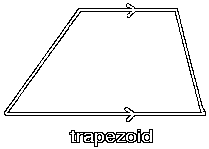The trapezoid is a quadrilateral with one pair of parallel sides.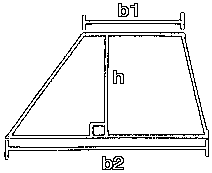The parallel sides of a trapezoid are called the bases, here symbolized by b1 and b2.

The height of the trapezoid is the perpendicular distance between the bases, here symbolized by h.

The area of the trapezoid is equal to the average of the bases times the height. So, you add the two bases, divide by 2, and then multiply by the height. This would be:

area = ((b1 + b2) / 2)h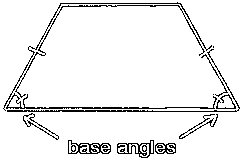If the two sides which are not parallel have equal lengths, then the trapezoid is called an isosceles trapezoid. The base angles in an isosceles trapezoid are equal in measurement.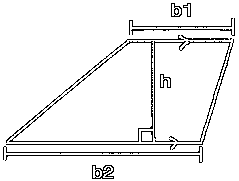Another example of a trapezoid.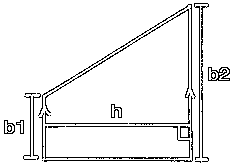Yet another example of a trapezoid.

Custom Search